# Hungarian Algorithm for Assignment Problem | Set 1 (Introduction)

• Difficulty Level : Hard
• Last Updated : 04 Mar, 2020

Let there be n agents and n tasks. Any agent can be assigned to perform any task, incurring some cost that may vary depending on the agent-task assignment. It is required to perform all tasks by assigning exactly one agent to each task and exactly one task to each agent in such a way that the total cost of the assignment is minimized.

Example: You work as a manager for a chip manufacturer, and you currently have 3 people on the road meeting clients. Your salespeople are in Jaipur, Pune and Bangalore, and you want them to fly to three other cities: Delhi, Mumbai and Kerala. The table below shows the cost of airline tickets in INR between the cities:

Attention reader! Don’t stop learning now. Get hold of all the important DSA concepts with the DSA Self Paced Course at a student-friendly price and become industry ready.  To complete your preparation from learning a language to DS Algo and many more,  please refer Complete Interview Preparation Course.

In case you wish to attend live classes with experts, please refer DSA Live Classes for Working Professionals and Competitive Programming Live for Students.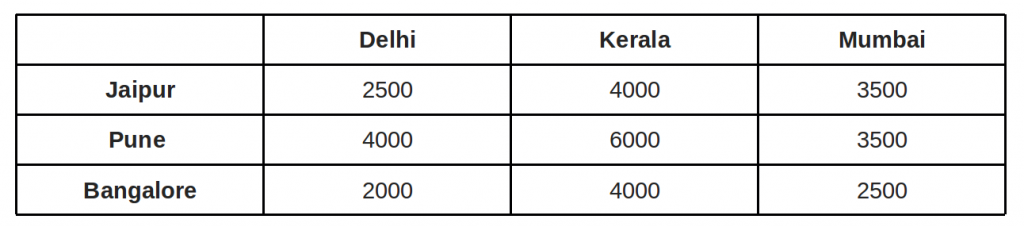The question: where would you send each of your salespeople in order to minimize fair?

Possible assignment: Cost = 11000 INR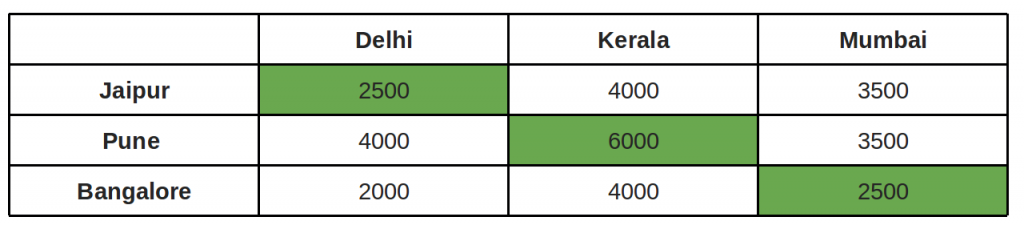Other Possible assignment: Cost = 9500 INR and this is the best of the 3! possible assignments.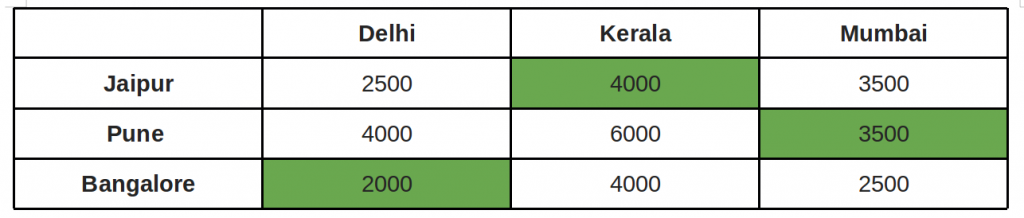Brute force solution is to consider every possible assignment implies a complexity of Ω(n!).

The Hungarian algorithm, aka Munkres assignment algorithm, utilizes the following theorem for polynomial runtime complexity (worst case O(n3)) and guaranteed optimality:
If a number is added to or subtracted from all of the entries of any one row or column of a cost matrix, then an optimal assignment for the resulting cost matrix is also an optimal assignment for the original cost matrix.

We reduce our original weight matrix to contain zeros, by using the above theorem. We try to assign tasks to agents such that each agent is doing only one task and the penalty incurred in each case is zero.

Core of the algorithm (assuming square matrix):

1. For each row of the matrix, find the smallest element and subtract it from every element in its row.
2. Do the same (as step 1) for all columns.
3. Cover all zeros in the matrix using minimum number of horizontal and vertical lines.
4. Test for Optimality: If the minimum number of covering lines is n, an optimal assignment is possible and we are finished. Else if lines are lesser than n, we haven’t found the optimal assignment, and must proceed to step 5.
5. Determine the smallest entry not covered by any line. Subtract this entry from each uncovered row, and then add it to each covered column. Return to step 3.

Explanation for above simple example:

```
Below is the cost matrix of example given in above diagrams.
2500  4000  3500
4000  6000  3500
2000  4000  2500

Step 1: Subtract minimum of every row.
2500, 3500 and 2000 are subtracted from rows 1, 2 and
3 respectively.

0   1500  1000
500  2500   0
0   2000  500

Step 2: Subtract minimum of every column.
0, 1500 and 0 are subtracted from columns 1, 2 and 3
respectively.

0    0   1000
500  1000   0
0   500  500

Step 3: Cover all zeroes with minimum number of
horizontal and vertical lines.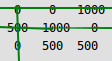Step 4:  Since we need 3 lines to cover all zeroes,
we have found the optimal assignment.
2500  4000  3500
4000  6000  3500
2000  4000  2500

So the optimal cost is 4000 + 3500 + 2000 = 9500
```

An example that doesn’t lead to optimal value in first attempt:
In the above example, the first check for optimality did give us solution. What if we the number covering lines is less than n.

```
cost matrix:
1500  4000  4500
2000  6000  3500
2000  4000  2500

Step 1: Subtract minimum of every row.
1500, 2000 and 2000 are subtracted from rows 1, 2 and
3 respectively.

0    2500  3000
0    4000  1500
0    2000   500

Step 2: Subtract minimum of every column.
0, 2000 and 500 are subtracted from columns 1, 2 and 3
respectively.

0     500  2500
0    2000  1000
0      0      0

Step 3: Cover all zeroes with minimum number of
horizontal and vertical lines.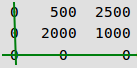Step 4:  Since we only need 2 lines to cover all zeroes,

Step 5:  We subtract the smallest uncovered entry
from all uncovered rows. Smallest entry is 500.
-500    0   2000
-500  1500   500
0     0      0

Then we add the smallest entry to all covered columns, we get
0     0   2000
0   1500   500
500    0      0

lines. and go to Step 4:. Since we need 3 lines to
cover, we found the optimal solution.
1500  4000  4500
2000  6000  3500
2000  4000  2500

So the optimal cost is 4000 + 2000 + 2500 = 8500
```

In the next post, we will be discussing implementation of the above algorithm. The implementation requires more steps as we need to find minimum number of lines to cover all 0’s using a program.